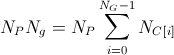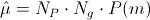io.jenetics

## Class Mutator<G extends Gene<?,G>,C extends Comparable<? super C>>

• All Implemented Interfaces:
Alterer<G,C>
Direct Known Subclasses:
GaussianMutator, SwapMutator

public class Mutator<G extends Gene<?,G>,C extends Comparable<? super C>>
extends AbstractAlterer<G,C>
This class is for mutating a chromosomes of an given population. There are two distinct roles mutation plays
• Exploring the search space. By making small moves mutation allows a population to explore the search space. This exploration is often slow compared to crossover, but in problems where crossover is disruptive this can be an important way to explore the landscape.
• Maintaining diversity. Mutation prevents a population from correlating. Even if most of the search is being performed by crossover, mutation can be vital to provide the diversity which crossover needs.

The mutation probability is the parameter that must be optimized. The optimal value of the mutation rate depends on the role mutation plays. If mutation is the only source of exploration (if there is no crossover) then the mutation rate should be set so that a reasonable neighborhood of solutions is explored.

The mutation probability P(m) is the probability that a specific gene over the whole population is mutated. The number of available genes of an population iswhere NP is the population size, Ng the number of genes of a genotype. So the (average) number of genes mutated by the mutation isSince:
1.0
Version:
4.0
Author:
Franz Wilhelmstötter

• ### Fields inherited from class io.jenetics.AbstractAlterer

_probability
• ### Fields inherited from interface io.jenetics.Alterer

DEFAULT_ALTER_PROBABILITY
• ### Constructor Summary

Constructors
Constructor and Description
Mutator()
Default constructor, with probability = 0.01.
Mutator(double probability)
Construct a Mutation object which a given mutation probability.
• ### Method Summary

All Methods
Modifier and Type Method and Description
AltererResult<G,C> alter(Seq<Phenotype<G,C>> population, long generation)
Concrete implementation of the alter method.
protected MutatorResult<Chromosome<G>> mutate(Chromosome<G> chromosome, double p, Random random)
Mutates the given chromosome.
protected MutatorResult<Genotype<G>> mutate(Genotype<G> genotype, double p, Random random)
Mutates the given genotype.
protected G mutate(G gene, Random random)
Mutates the given gene.
protected MutatorResult<Phenotype<G,C>> mutate(Phenotype<G,C> phenotype, long generation, double p, Random random)
Mutates the given phenotype.
String toString()
• ### Methods inherited from class io.jenetics.AbstractAlterer

equals, getProbability, hashCode
• ### Methods inherited from class java.lang.Object

clone, finalize, getClass, notify, notifyAll, wait, wait, wait
• ### Methods inherited from interface io.jenetics.Alterer

andThen, compose, of
• ### Constructor Detail

• #### Mutator

public Mutator(double probability)
Construct a Mutation object which a given mutation probability.
Parameters:
probability - Mutation probability. The given probability is divided by the number of chromosomes of the genotype to form the concrete mutation probability.
Throws:
IllegalArgumentException - if the probability is not in the valid range of [0, 1]..
• #### Mutator

public Mutator()
Default constructor, with probability = 0.01.

© 2007-2018 Franz Wilhelmstötter  (2018-10-28 17:23)